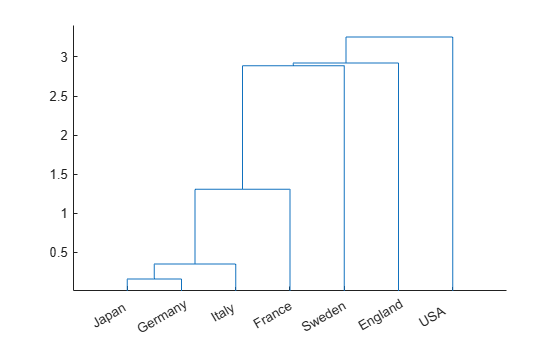Documentation

manovacluster

Dendrogram of group mean clusters following MANOVA

Syntax

manovacluster(stats)
manovacluster(stats,method)
H = manovacluster(stats,method)

Description

manovacluster(stats) generates a dendrogram plot of the group means after a multivariate analysis of variance (MANOVA). stats is the output stats structure from manova1. The clusters are computed by applying the single linkage method to the matrix of Mahalanobis distances between group means.

See dendrogram for more information on the graphical output from this function. The dendrogram is most useful when the number of groups is large.

manovacluster(stats,method) uses the specified method in place of single linkage. method can be one of these values, which identify the methods used to create the cluster hierarchy. (See linkage for additional information.)

MethodDescription

'single'

Shortest distance (default)

'complete'

Largest distance

'average'

Average distance

'centroid'

Centroid distance

'ward'

Incremental sum of squares

H = manovacluster(stats,method) returns a vector of handles to the lines in the figure.

Examples

collapse all

Load the sample data.

Define the variable matrix.

X = [MPG Acceleration Weight Displacement];

Perform one-way MANOVA to compare the means of MPG, Acceleration, Weight,and Displacement grouped by Origin.

[d,p,stats] = manova1(X,Origin);

Create a dendrogram plot of the group means.

manovacluster(stats)